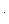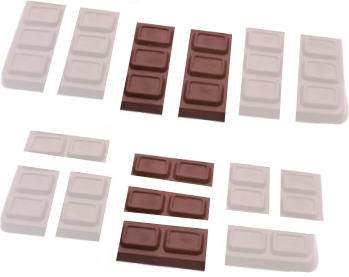# Equivalent Fractions

### What are equivalent fractions?

By

If I want half a pizza, but the pizza is cut into four pieces, how many should I eat?To most people, getting one half of a pizza is the same as getting two quarters of the pizza. At least, we get the same amount of pizza either way! This is what we mean when we say
The fractionsandare equivalent fractions
The word equivalent means, literally, "same value". So equivalent fractions are ones with the same value as numbers or amounts.

Let's see another example. Suppose there is a chocolate bar divided into 6 parts, and I get two, so my share is two sixths.Now imagine, instead, the bar was divided into nine pieces, and I got three, well, now I have three ninths, but I still have the same amount of chocolate. Therefore,
The fractionsandare equivalent fractions
This is what it means for fractions to be equivalent.

### To test if fractions are equivalent

I can think of three methods for doing this.

#### The first method for equivalent fractions

The first method to test if fractions are equivalent fractions is to simplify the fractions. For example, to answer this question :
Which of the fractionsandare equivalent?
we can start by simplifying each fraction. For this problem,simplifies to,simplifies to,
butsimplifies to.
Because 18/30 and 24/40 become exactly the same when properly simplified, they are equivalent fractions. However, 20/35 simplifies to something different.
The key here is
Equivalent fractions give the same answer when you simplify them
It's important to simplify them properly though! If you simplified 18/30  to  3/5  and 24/40 to 6/10 , you couldn't say they were not equivalent - you haven't finished simplifying 24/40...

#### The second method for equivalent fractions

If you put equivalent fractions over a common denominator, the results will be the same. Let's try this with the example above.
If we putandover a common denominator,
we getandThese are different, so the fractions are not equivalent. However,
if we putandover a common denominator,
we getandThese are the same! So, these two fractions are equivalent. The principle here is
Equivalent fraction become the same when you put them over a common denominator

#### The third method for equivalent fractions

If you are uncomfortable with the two methods above, but you like multiplying, you can try this method
• Multiply the denominator of one fraction by the numerator of the other.
• Then multiply the numerator of the first fraction by the denominator of the other.
• If these two calculations give the same answer, then the fractions are equivalent fractions. Otherwise, they are not.
For example, withand• 30 times 20 is 600, but
• 18 times 35 is 630, so
• These fractions are not equivalent
Now, let's tryandHere,
• 30 times 24 is 720, and
• 18 times 40 is also 720, so
• These fractions are equivalent!

If you'd like some practice working out equivalent fractions, be sure to check out the online Equivalent Fractions Quiz, or the Equivalent Fractions Worksheets on this site. Or would you like an infinite supply of Equivalent Fractions Examples? If you do the quiz or the worksheets, don't use the Equivalent Fractions Calculator to cheat!